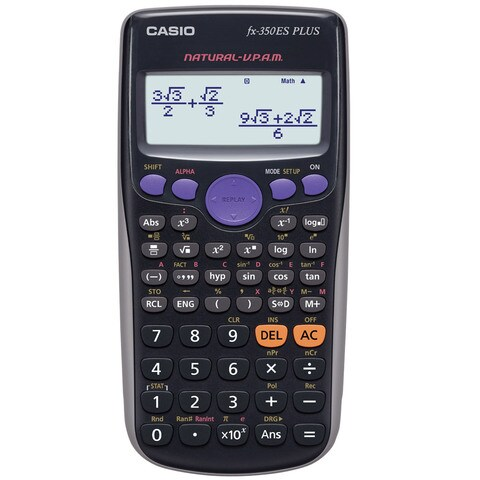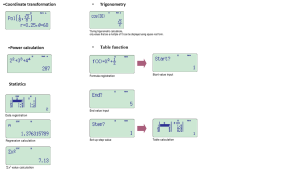# Casio Scientific Calculator – FX350ESPLUS

• Basic Calculation
• Bracket Calculation
• Fraction Calculation
• Degree, Minute, Secound (Sexagesimal) Calculations
• Reciprocal Calculation
• Percent Calculation (scientific calculator function)
• Factorial Function
• π
• Irrational number calcuation
• Rounding Function

AED 65.00

Item will be shipped in 1-3 business days
Casio FX-350ES Plus Scientific Calculator 10-digit mantissa + 2-digit exponential display. New functions-Prime factorizations and Random integers. Standard functions-Fraction calculations,Combination and permutation Statistics (List-based STAT data editor, standard deviation, regression analysis) 9 variables and Table function.Comes with new slide-on hard case.• Fraction calculations
• Combination and permutation
• Statistics (List-based STAT data editor, standard deviation, regression analysis)
• 9 variables
• Table function
• Comes with new slide-on hard caseSKU: FX350ESPLUS2 Category:
Product features
Non Programmable
Non Graphing
Number of Functions : 252
Product type
Non Programmable
Non Graphing
Number of digits
10 + 2 digits
Liquid crystal display
Natural textbook display
Dot matrix display
Key characteristics
Plastic keys
Key functions
Negative Sign
Power Off
Memory
Variables: 9 (A,B,C.D.E.F.X.Y,M)
Independent Memory (M / M+ / M-)
Memory Protection
Reset Function
Power supply
LR44×1
Approximate battery life Main 3 years
Auto Power Off
Size (D × W × H)
16.15 × 7.7 × 1.11 cm
Weight
0.95 g

## Based on 0 reviews

0.0 overall
0
0
0
0
0

There are no reviews yet.0 out of 5
Industrial Area 6, Backside of Macro, Sharjah United Arab Emirates - 33108
+971 56 685 8490

## General Inquiries

There are no inquiries yet.